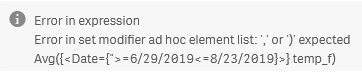App Development

Announcements
QlikWorld 2022, LIVE in Denver CO., May 16-19, 2022. REGISTER NOW TO RECEIVE EARLY BIRD PRICING
cancel
Showing results for
Did you mean:Creator III

Use an island table to look up a date range, and pass that to a chart?

I have a weather app with data imported from IOT devices. I would like to know if it would be possible to add an island table that contains work order numbers, start dates, and end dates.  The idea is that I could provide a drop-down list of work orders and the user could select one, which would pull up the start and end dates for that work order which would be used to define a date range to filter the chart.  Would this be possible? If so, could you provide an example?

Thank you.

1 Solution

Accepted SolutionsMVP

Hi, yes, this can be done but you need to add set analysis in all expressions, something like:

Sum({<Date={">=\$(=Date(Min(StartDate)))<=\$(=Date(Min(EndDate)))}>} ValueToSumInChart)

StartDate and EndDate are the fields filtered in island table.

Date is the field in the fact table used to filter the chart

4 RepliesMVP

Hi, yes, this can be done but you need to add set analysis in all expressions, something like:

Sum({<Date={">=\$(=Date(Min(StartDate)))<=\$(=Date(Min(EndDate)))}>} ValueToSumInChart)

StartDate and EndDate are the fields filtered in island table.

Date is the field in the fact table used to filter the chartCreator III
Author

Hi @rubenmarin .  Thanks for your suggestion but I'm getting a syntax error; can you take a look? I just copy/pasted your expression and the syntax error shows up whether I keep your field names or replace with my own, so it seems like a true syntax error and not related to the field names.MVP

Hi, that means that the values inside the \$( can't be calculated, check field names, or extract that part to a new expression to debug it: =Date(Min(StartDate))

Confirm the field with the start dates and use that field name instead of Startdate, same for EndDateCreator III
Author

@rubenmarinI found the error; the closing quotes were missing on the right side of the expression.Here's the final version and it is working now.  Thank you.

Avg({<Date={">=\$(=Date(Min(PLANTDT)))<=\$(=Date(Min(CLOSEDT)))"}>} temp_f)Tags
Community Browser